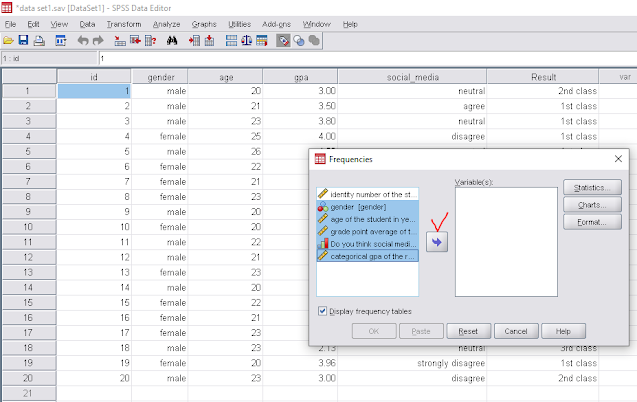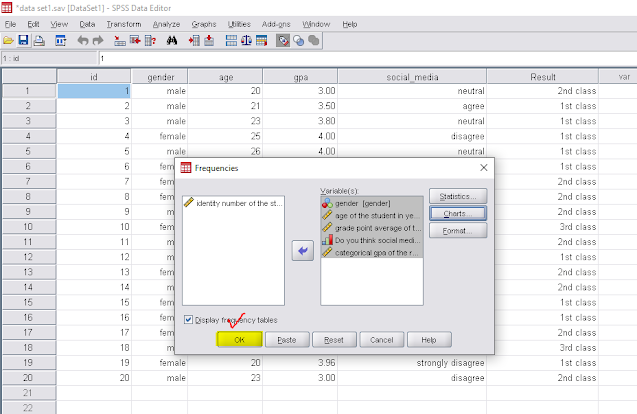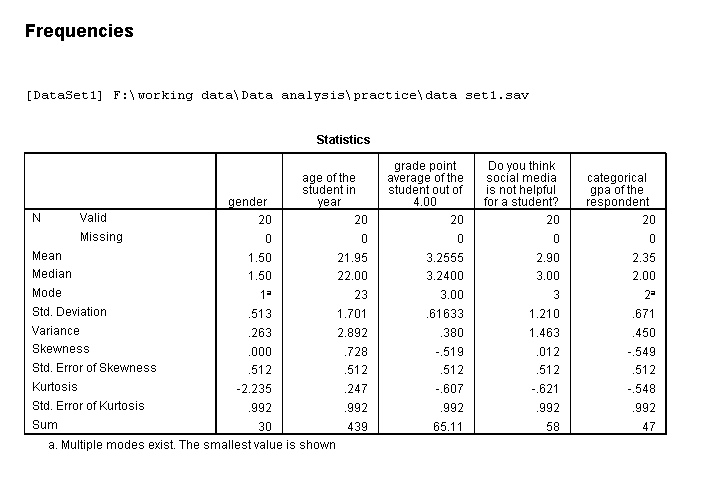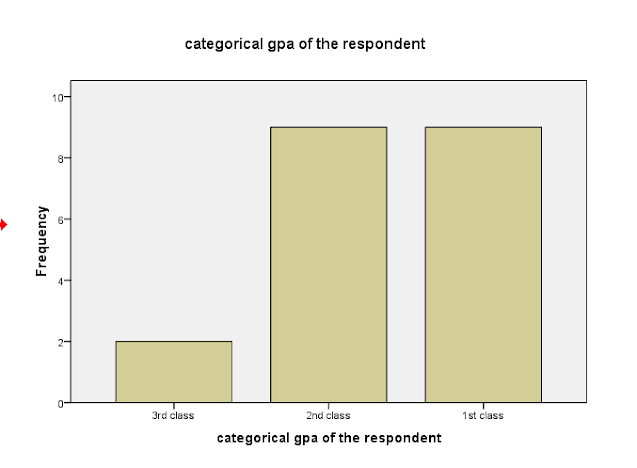# Univariate analysis using spss (data analysis part-9)

Three different types of procedures can be used to analyze data in statistics. These include univariate, bivariate, and multivariate analysis. The amount of variables and the type of data determine which data analysis method is used. Additionally, we have to consider the objectives of the statistical analysis. We can easily conduct univariate analysis using SPSS, Stata, R and Excel software. The univariate analysis’s details are described in this article.

## What is univariate analysis?

Univariate analysis is a simple and fundamental type of statistical data analysis where only one variable is used. There is no relationship between a cause and an effect in this data set because there is just one variable. It is mostly used to describe data. After summarizing the data, the analysis will look for patterns.

### Types

There are some essential and popular univariate analysis,

• Summary Statistics: It is the most popular method for conducting univariate analysis. Measure of dispersion and measure of central tendency are the common technique of summary statistics. The range, standard deviation, variance, and interquartile range are some examples of measure of dispersion and mean, median, mode are basic measures of central tendency. We also used frequency distribution table which tells us the number of times for the occurrence of an event.
• Bar chart: Rectangular bars are used to describe the bar chart. Various categories will be compared in the graph. These could either be plotted horizontally or vertically on the graph. The bar graph explains and compares the data set.
• Pie Chart: The pie chart displays the data in a circular format. The graph is divided into slices where each slice is proportional to the fraction of the complete category. So each slice of the pie chart is relative to categories size. The sum of all slice of the pie chart must be 100 percent.

We also use frequency polygon and histogram to describe univariate characteristics of a variable.

### Univariate analysis using SPSS

We conduct Univariate analysis using SPSS to find the characteristics of the variable. SPSS is a simple statistical analytics tool which is very easy to use and maintain. It is the analysis of one random variable such as descriptive statistics (mean, variance etc.).
We have the following data set with the several highlighted variable.

Now we have to go to the following steps.Data set

Let we have five variables named gender, age, gpa, social_media and result. If we analyze each of the variable separately then its called univariate analysis. we can find the mean, variance and other descriptive statistics using univariate analysis. For univariate analysis first we have to go to–

1.Analyze →→Descriptive statistics→→ Frequencies

Now we have to select all the variable and taking them into the empty box named Variable(s).Frequencies

2.we have to click on StatisticsFrequencies

3. Select all the required area such as mean, median, standard deviation etc and click on Continue.Frequencies statistics

4. if we want the graph such as bar chart, pie chart then click on Charts and select the chart types and click on continue.Frequencies chart

5. click on Ok .Select OK

6. Now we can see the output window as following.FrequenciesBar chart